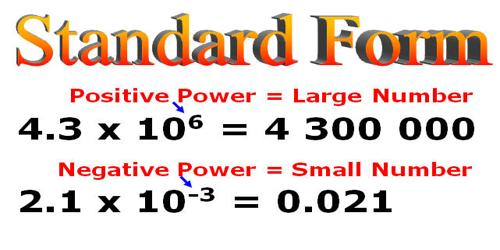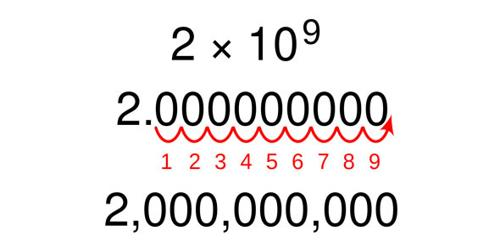Mathematic

# Comparing Scientific and Standard Notation NumbersComparing Scientific and Standard Notation Numbers

Scientific notation is used to express very large or very small numbers. A number in scientific notation is written as the product of a number (integer or decimal) and a power of 10. This number is always 1 or more and less than 10.

Scientific Notation

Imagine that you are a scientist working for the government. Your job is to look through test data from other researchers. Right now you are working on a rather important project. And so, you are looking at a piece of paper with a lot of numbers written in scientific notation, a special way to write numbers. These numbers are written in scientific notation because they are either very large or very small.

Remember that your numbers written in scientific notation all have a multiplication by 10 to a power. So, some of the numbers you are seeing are numbers such as 2.3 * 1012, 4.67 * 1054, 3.12 * 10-9, etc. Your job is to put these numbers in order so that you can better understand the data.

For example, there are approximately 6,000,000,000 humans on earth. This number could be written in scientific notation as 6×109. The number 6,000,000,000 is equivalent to 6*1,000,000,000. The number 1,000,000,000 is equivalent to 109 or 10*10*10*10*10*10*10*10*10.Two numbers written in scientific notation can be compared. The number with the larger power of 10 is greater than the number with the smaller power of 10. If the powers of ten are the same then the number with the larger factor is the larger number

For example, 3.4×107 is greater than 3.4×104 (It might be a little easier to see if we convert them to standard form: 34,000,000 and 34,000, but it’s much faster to simply look at the exponents).

A number can be converted to scientific notation by increasing the power of ten by one for each place the decimal point is moved to the left. In the example above, the decimal point was moved 9 places to the left to form a number more than 1 and less than 10.

Scientific notation numbers may be written in different forms. The number 6×109 could also be written as 6e+9. The +9 indicates that the decimal point would be moved 9 places to the right to write the number in standard form.

How to Compare Numbers

What you need to do is to compare these numbers to each other. You need to be able to determine which number is larger and which is smaller. Let me first give you the steps you need to take to do so, and then we will look at a couple of examples.

There are only two steps you need to take. The first is to look at the power and compare these numbers together. If one is larger or smaller, then you already know which number is larger or smaller. The larger the exponent, the larger the number is. And, vice versa, the smaller the exponent, the smaller the number is. If your powers are the same, then you move on to the second step. If they are not the same, then you are done comparing the numbers.

The second step is to look at the number before the multiplication by 10 if the powers are the same. Treat these like you would normal numbers. If one is larger, then that number is larger, and if one is smaller, then that number is smaller. Let’s look at a couple of examples now to see how it all works.

Example

Two numbers written in scientific notation can be compared. The number with the larger power of 10 is greater than the number with the smaller power of 10. If the powers of ten are the same then the number with the larger factor is the larger number.

For example, 3.4×107 is greater than 3.4×104. The number 3.4×107 is greater than 3.1×107.

Numbers written in standard form can be compared to numbers written in scientific notation by converting one number to the other format.

For example, to compare 3.4×107 and 4,500,000 convert 3.4×107 to 34,000,000 or convert 4,500,000 to 4.5×106 before comparing them.

Information Source: